# Problem: Rotational motion with a constant nonzero acceleration is not uncommon in the world around us. For instance, many machines have spinning parts. When the machine is turned on or off, the spinning parts tend to change the rate of their rotation with virtually constant angular acceleration. Many introductory problems in rotational kinematics involve motion of a particle with constant, nonzero angular acceleration. The kinematic equations for such motion can be written as and .Here, the symbols are defined as follows: is the angular position of the particle at time . is the initial angular position of the particle. is the angular velocity of the particle at time . is the initial angular velocity of the particle. is the angular acceleration of the particle. is the time that has elapsed since the particle was located at its initial position.A)Which of the following equations is not an explicit function of time ? Keep in mind that an equation that is an explicit function of time involves  as a variable.a) b) c) B)In the equation , what does the time variable  represent?Choose the answer that is always true. Several of the statements may be true in a particular problem, but only one is always true.a) the moment in time at which the angular velocity equals b) the moment in time at which the angular velocity equals .c) the time elapsed from when the angular velocity equals  until the angular velocity equals .

###### FREE Expert Solution

(A)

Explicit functions of time have a variable t.

Implicit functions depend on a variable which is not directly visible in the equation.###### Problem Details

Rotational motion with a constant nonzero acceleration is not uncommon in the world around us. For instance, many machines have spinning parts. When the machine is turned on or off, the spinning parts tend to change the rate of their rotation with virtually constant angular acceleration. Many introductory problems in rotational kinematics involve motion of a particle with constant, nonzero angular acceleration. The kinematic equations for such motion can be written as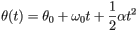and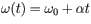.

Here, the symbols are defined as follows:

•is the angular position of the particle at time.
•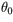is the initial angular position of the particle.
•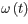is the angular velocity of the particle at time.
•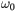is the initial angular velocity of the particle.
•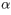is the angular acceleration of the particle.
•is the time that has elapsed since the particle was located at its initial position.

A)

Which of the following equations is not an explicit function of time? Keep in mind that an equation that is an explicit function of time involvesas a variable.

a)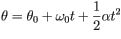b)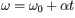c)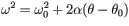B)

In the equation, what does the time variablerepresent?

Choose the answer that is always true. Several of the statements may be true in a particular problem, but only one is always true.

a) the moment in time at which the angular velocity equalsb) the moment in time at which the angular velocity equals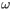.

c) the time elapsed from when the angular velocity equalsuntil the angular velocity equals.